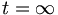# Kirchhoff problem 2 solution

At t=0, the capacitor acts like a short circuit and the inductor acts like an open circuit, and we can simply the cicuit into:

VR1 = 6.818V

VR2 = 0V

VR3 = VR4 = 8.182V

IR1 = 13.64mA

IR2 = 0A

IR3 = 5.455mA

IR4 = 8.182mA

At$t=\infty$, the capacitor acts like an open circuit and the inductor acts like a short circuit. We can then simplify the circuit:

VR1 = 9V

VR2 = 36V

VR3 = 0V

VR4 = 6V

IR1 = 18mA

IR2 = 12mA

IR3 = 0A

IR4 = 6mA